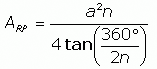SEARCH HOMEMath Central Quandaries & QueriesQuestion from molly, a student: in the formula to find the area of any regular polygon how do you figure out 4tan part of the formulaMolly,

I think the expression you are using iswhere a is the length of a side and n is the number of sides. To evaluate the denominator use the tan button on your calculator. For example for an octagon where n = 8, 360o/(2n) = 360o/16 = 22.5o. Make sure your calculator is in degree mode not radian mode, and evaluate tan(22.5o). I got tan(22.5o) = 0.414214. Thus the denominator is 4 tan(22.5) = 4 × 0.414214 = 1.6569.

I hope that helps,
PennyMath Central is supported by the University of Regina and The Pacific Institute for the Mathematical Sciences.